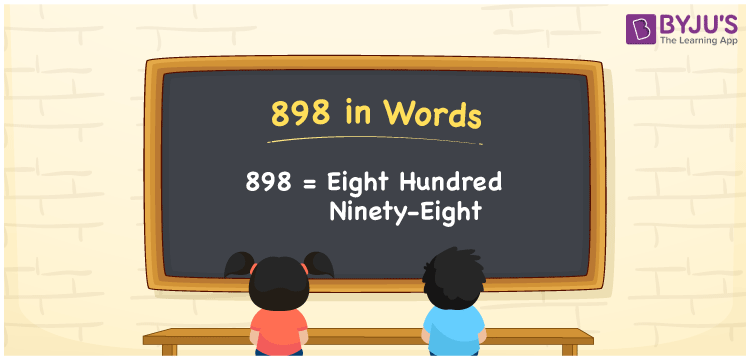# 898 in Words

898 in words is written as Eight hundred ninety-eight. In both the International System of Numerals and the Indian System of Numerals, 898 is written as Eight hundred ninety-eight. The number 898 is a Cardinal Number as it defines some quantity. For example, “this item costs 898 rupees”.

 898 in Words Eight hundred ninety-eight Eight hundred ninety-eight in Number 898

## 898 in English Words

We write 898 in English Words using the letters of English alphabets. Therefore, we read 898 in English as “Eight hundred ninety-eight”## How to Write 898 in Words?

To write 898 in words, we shall use the place value chart. In the place value chart, write 8 in the hundreds, 9 in the tens, and 8 in the ones, respectively. Now let us make a place value chart to write the number 898 in words.

 Hundreds Tens Ones 8 9 8

Thus, we can write the expanded form as

8 × Hundred + 9 × Ten + 8 × One

= 8 × 100 + 9 × 10 + 8 × 1

= 800 + 90 + 8

= 898

= Eight hundred ninety-eight.

898 is a natural number, the successor of 897 and the predecessor of 899.

898 in words – Eight hundred ninety-eight

• Is 898 an odd number? – No
• Is 898 an even number? – Yes
• Is 898 a perfect square number? – No
• Is 898 a perfect cube number? – No
• Is 898 a prime number? – No
• Is 898 a composite number? – Yes

## Frequently Asked Questions on 898 in Words

Q1

### How to write 898 in words?

898 in words is written as Eight hundred ninety-eight.
Q2

### How to write 898 in words in the International and Indian System of Numerals?

In both the system of numerals, 898 is written as Eight hundred ninety-eight.
Q3

### What is the successor of 898?

The successor of 898 is 899.